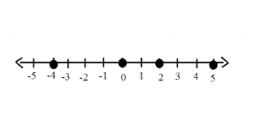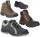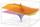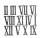# Pair of numbers

What is the value of the smaller of a pair of numbers for which their sum is 78, and their division quotients are 0.3?

a =  18

### Step-by-step explanation:Did you find an error or inaccuracy? Feel free to write us. Thank you!Tips to related online calculators
Do you have a linear equation or system of equations and looking for its solution? Or do you have a quadratic equation?

## Related math problems and questions:

• The ratioThe ratio of two numbers is 4, and their sum is 75. Determine both numbers.
• The sumThe sum of five consecutive odd numbers is 75. Find out the sum of the second and fourth of them.
• Two numbersThe difference between the two numbers is 74. If we divide a larger number by a smaller one, we get a quotient 7 and the rest of 2. Determine both numbers.
• Unknown numberIf I reduced the sum of the numbers 70 and the unknown number three times, I would get 100. what is the unknown number?
• ShoesJoseph had one pair of sandals, one pair of hiking shoes, 1 pair of climbing shoes, one pair of church shoes. How many pieces of shoes had together?
• Sum of three numbersThe sum of three numbers from which second number is 20% smaller than the first number and the third number is 25% smaller than the second number is 96. Determine this numbers.
• AS sequenceIn an arithmetic sequence is given the difference d = -3 and a71 = 455. a) Determine the value of a62 b) Determine the sum of 71 members.
• Sum 1-6Find the sum of the geometric progression 3, 15, 75,… to six terms.
• Sum-logThe sum of two numbers is 32, the sum of their logarithms (base 10) is 2.2. Determine these numbers.
• The number 72The number 72 increase by 25%. By how much % will you have to reduce the number you created to get the number 72 again?
• The difference 2The difference between the two numbers is 25. The smaller number is 1/6th of the larger number. What is the value of the smaller number?
• Four multiplesFour multiples of 6 he writes the following 12  24  56  72, which is correct?
• The sum 8The sum of two numbers is 21. If three times the smaller numbers is two less than twice the larger number, find the two numbers.
• The quotientThe quotient of g and 55 is the same as 279. What is g?
• Bricks pyramidHow many 50cm x 32cm x 30cm brick needed to built a 272m x 272m x 278m pyramid?
• Four integersFnd four consecutive integers so that the product of the first two is 70 times smaller than the product of the next two.
• Unknown number4/5 of a number is 276. what is 2/3 of the same number?